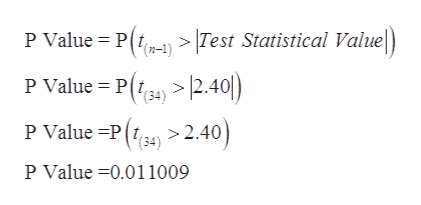# Members of the school committee for a large city claim that the population mean class size of a middle school class within the district is exactly 20 students. Karla, the superintendent of schools for the city, thinks the population mean is less. She selects a random sample of 35 middle school classes across the city. The sample mean is 18.5 students with a sample standard deviation of 3.7 students. The test statistic t for a hypothesis test of H0:μ=20 versus Ha:μ<20 is t≈−2.40. If 0.01<p-value<0.025 and the level of significance is α=0.05, which of the following statements are accurate for this hypothesis test to evaluate the claim that the true population mean class size of a middle school class within the district is less than 20 students?Select all that apply:A) Reject the null hypothesis that the true population mean class size of a middle school class within the district is equal to 20 students.B) Fail to reject the null hypothesis that the true population mean class size of a middle school class within the district is equal to 20 students.C) There is not enough evidence at the α=0.05 level of significance to suggest that the true population mean class size of a middle school class within the district is less than 20 students.D) There is enough evidence at the α=0.05 level of significance to support the claim that the true population mean class size of a middle school class within the district is less than 20 students.

Question
124 views

Members of the school committee for a large city claim that the population mean class size of a middle school class within the district is exactly 20 students. Karla, the superintendent of schools for the city, thinks the population mean is less. She selects a random sample of 35 middle school classes across the city. The sample mean is 18.5 students with a sample standard deviation of 3.7 students. The test statistic t for a hypothesis test of H0:μ=20 versus Ha:μ<20 is t≈−2.40. If 0.01<p-value<0.025 and the level of significance is α=0.05, which of the following statements are accurate for this hypothesis test to evaluate the claim that the true population mean class size of a middle school class within the district is less than 20 students?

Select all that apply:

A) Reject the null hypothesis that the true population mean class size of a middle school class within the district is equal to 20 students.

B) Fail to reject the null hypothesis that the true population mean class size of a middle school class within the district is equal to 20 students.

C) There is not enough evidence at the α=0.05 level of significance to suggest that the true population mean class size of a middle school class within the district is less than 20 students.

D) There is enough evidence at the α=0.05 level of significance to support the claim that the true population mean class size of a middle school class within the district is less than 20 students.

check_circle

Step 1

According to the question,

H0: μ=20 versus Ha: μ<20

We have n = 35

Level of significance = 0.05

t = -2.40

Step 2
• Since P values (0.011009) is less than level of significance (0.05)
• Which shows that we have enough evidence to reject the null hypothesis and support the claim that mean class size of a middle school class within the district is less than ...help_outlineImage TranscriptioncloseP Value P(> |Test Statistical Value P Value P(>2.40|) (34) P Value =P > 2.40) P Value 0.01 1009 fullscreen

### Want to see the full answer?

See Solution

#### Want to see this answer and more?

Solutions are written by subject experts who are available 24/7. Questions are typically answered within 1 hour.*

See Solution
*Response times may vary by subject and question.
Tagged in

### Hypothesis Testing